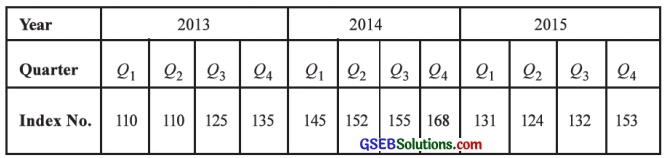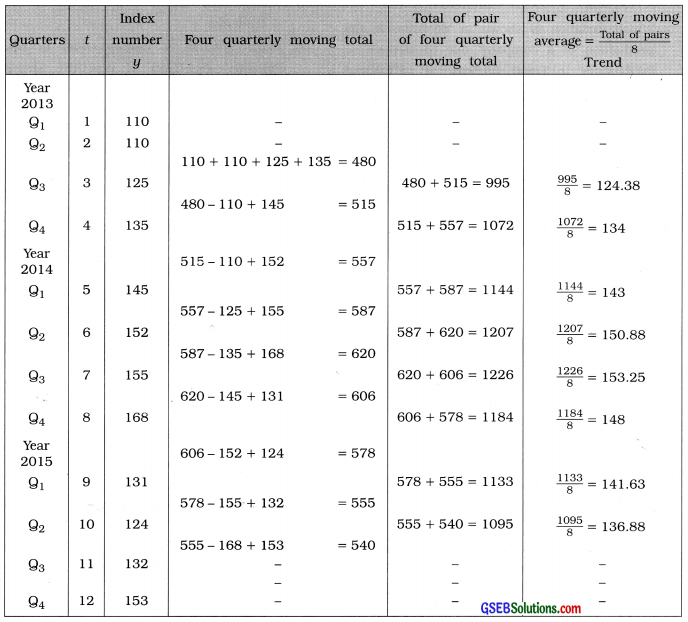# GSEB Solutions Class 12 Statistics Part 1 Chapter 4 Time Series Ex 4.3

Gujarat Board Statistics Class 12 GSEB Solutions Part 1 Chapter 4 Time Series Ex 4.3 Textbook Exercise Questions and Answers.

## Gujarat Board Textbook Solutions Class 12 Statistics Part 1 Chapter 4 Time Series Ex 4.3

Question 1.
Find the trend by three yearly moving averages from the following data about the sales (in ten lakh ₹) of a company: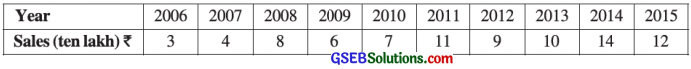Here, n = 10; So t = 1, 2, 3, 10; y = Sales
The table for calculating three yearly moving average is prepared as follows: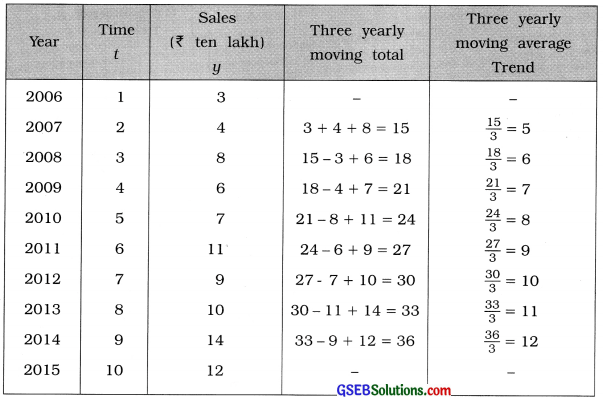Question 2.
The average monthly closing prices of shares of a company in the year 2016 are given in the following table. Find the trend using four monthly moving averages.Here, n = 12 months; So t = 1, 2, 3, ………, 12; y = Share price
To calculate four monthly moving average, the table is prepared on follows:Question 3.
Find the trend using five yearly moving averages from the following data of profit (in lakh ₹) of a trader in different years: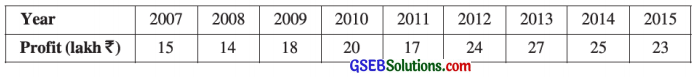Here, n = 9; So t = 1, 2, ……, 9; y = Profit
To calculate five yearly moving average the table is prepared as follows:Question 4.
The wholesale price index numbers for different quarters (9) of a year are obtained as follows. Find the trend by four quarterly moving averages.